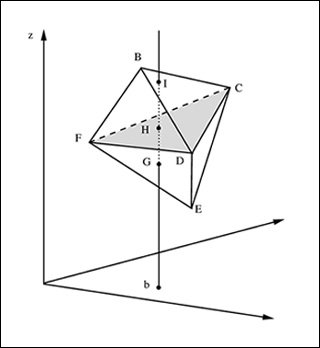6.251J | Fall 2009 | Graduate

# Introduction to Mathematical Programming

#### Course Description

This course is an introduction to linear optimization and its extensions emphasizing the underlying mathematical structures, geometrical ideas, algorithms and solutions of practical problems. The topics covered include: formulations, the geometry of linear optimization, duality theory, the simplex method, sensitivity …

## Course Info

##### Learning Resource Types
Problem Sets
Lecture NotesTopics in geometric programming are covered in lectures 2-4. (Courtesy of D. Bertsimas and J. N. Tsitsiklis, Introduction to Linear Optimization, Athena Scientific, 1997.)